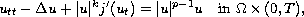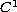Electron. J. Diff. Eqns., Vol. 2007(2007), No. 76, pp. 1-10.

### Blowup and asymptotic stability of weak solutions to wave equations with nonlinear degenerate damping and source terms Qingying Hu, Hongwei Zhang

Abstract:
This article concerns the blow-up and asymptotic stability of weak solutions to the wave equationwhere p›1 and j' denotes the derivative of aconvex and real value function j. We prove that every weak solution is asymptotically stability, for every m such that 0‹m‹1, p‹k+m and the the initial energy is small; the solutions blows up in finite time, whenever p›k+m and the initial data is positive, but appropriately bounded.

Editors note: A reader informed us that that parts of the introduction were copied from reference , without giving the proper credit. Also that the first statement in Lemma 4.3 maybe false; so that theorem 4.5 has not been proved. The authors agreed to post a new proof, if they succeed in proving the lemma.

Submitted February 27, 2007. Published May 22, 2007.
Math Subject Classifications: 35B40.
Key Words: Wave equation; degenerate damping and source terms; asymptotic stability; blow up of solutions.

Show me the PDF file (240K), TEX file, and other files for this article.Qingying Hu Department of Mathematics Henan University of Technology Zhengzhou 450052, China email: slxhqy@yahoo.com.cn Hongwei Zhang Department of Mathematics Henan University of Technology Zhengzhou 450052, China email: wei661@yahoo.com.cn